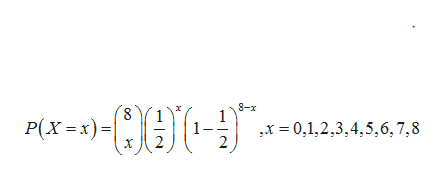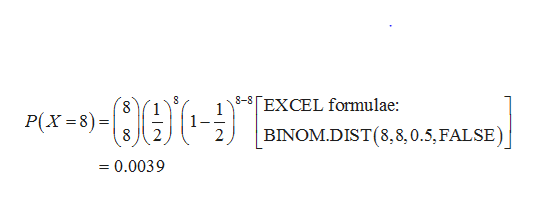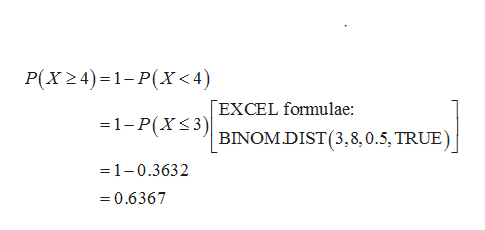Suppose you flip eight fair coins. What is the probability of flipping exactly eight heads?What is the probability of flipping at least four heads?What is the probability of flipping at most seven heads?What is the probability of flipping less than three heads?What is the probability of flipping more than one head?

Question
1. Suppose you flip eight fair coins.

1. What is the probability of flipping exactly eight heads?
2. What is the probability of flipping at least four heads?
3. What is the probability of flipping at most seven heads?
4. What is the probability of flipping less than three heads?
5. What is the probability of flipping more than one head?
Step 1

In a coin tossing the probability of getting a head and tail is equal and the corresponding probability of each is equal to ½. Here the eight coin are tossed. Let X be the probability of getting heads. Thus, it follows a binomial distribution with n=8 and p=1/2. That is,help_outlineImage Transcriptionclose8-x Р(х - х) - ,x 0,1,2,3,4,5,6,7,8 fullscreen
Step 2

1.Finding the probability of flipping exactly eight heads:

The probability of flipping exactly eight heads is 0.0039 and is obtained as follows:help_outlineImage Transcriptionclose8-8 [EXCEL formulae: Р(х -8)-D BINOM.DIST(8,8,0.5, FALSE) 2 =0.0039 fullscreen
Step 3

2.Finding the probability of flipping at least 4 heads:

The probability of flipping exactly...help_outlineImage TranscriptioncloseP(X24) 1-P(X<4) EXCEL formulae: =1-P(XS3) BINOMDIST(3,8,0.5, TRUE) =1-0.3632 =0.6367 fullscreen

Want to see the full answer?

See Solution

Want to see this answer and more?

Our solutions are written by experts, many with advanced degrees, and available 24/7

See Solution
Tagged in

Other